# Performance Examinations of Quadrotor with Sliding Mode Control-Neural Network on Various Trajectory and Conditions

Performance Examinations of Quadrotor with Sliding Mode Control-Neural Network on Various Trajectory and Conditions
Department of Physics Engineering, Sepuluh Nopember Institute of Technology (ITS), Surabaya, East Java 60111, Indonesia

Corresponding Author Email:
Page:
707-714
|
DOI:
https://doi.org/10.18280/mmep.090317
6 December 2021
|
Accepted:
13 June 2022
|
Published:
30 June 2022
| Citation

OPEN ACCESS

Abstract:

In this article, the performance of the sliding mode control (SMC) that is combined with the backpropagation neural network (NN) as the main control of quadrotor’s dynamic systems was examined on various trajectories and conditions, through numerical simulation. The simulation is conducted with three different trajectories in the absence and presence of the time-varying external disturbances that were adopted from previous studies. The time-varying external disturbances are implemented for the roll, pitch, yaw, and altitude movement simultaneously with the gain set up at the value of 0.8. The simulation results show that the SMC-NN scheme was able to control the quadrotor either in the absence or in the presence of time-varying external disturbances, for each trajectory without any chattering or vibration issues in the quadrotor’s dynamic system. It can be concluded that the SMC-NN is one of the control strategies that are appropriate for the mission with various conditions and circumstances.

Keywords:

backpropagation neural network, backstepping approach, Lyapunov function, quadrotor, robust control, sliding mode control

1. Introduction

Quadrotor Unmanned Aerial Vehicles (UAVs) have received increasing attention in the past decade, because of their special characteristics such as autonomous flight , low-cost, vertical takeoff/ landing ability, and onboard vision system , and their wide applications such as military [3-5], surveillance [6, 7], agriculture monitoring [8, 9], mitigation and disasters rescue , building exploration and information collection , and many more. These popularities are closely related to the performance of the flight controller implemented . A quadrotor is an aircraft with four rotors in a cross configuration, which has four inputs (roll, pitch, yaw, and throttle) and so defines the motion behaviors in the three-dimensional space by changing its propeller angular speeds .

Trajectory tracking control of quadrotors is a complex open problem as a control scheme for fully actuated systems cannot be applied to underactuated quadrotor systems directly, due to the six degrees of freedom (6-DoF) with only four control inputs. It is known that quadrotors have complex and highly coupled dynamics, under-actuated properties, and are very sensitive to unmodelled dynamics, parametric uncertainties, and external disturbances (e. g., payload variation, model uncertainty, and wind gusts) [12, 13]. These make flight control a very challenging task, especially for trajectory tracking control.

A lot of control strategies have been proposed to address the aforementioned problems. For instance, the classical PID (proportional-integral-derivative) linear controller was applied to control both the altitude and attitude of a quadrotor [13-16] and it shows acceptable performance. However, the accuracy seems to decrease as the operating condition changes. It is caused by the PID control parameters that are designed to work in specific conditions, for example, quadrotor with or without external payload, therefore applying the PID controller to the quadrotor limits its performance . Some other types of control algorithms have been proposed such as a linear quadratic regulator or gaussian (LQR / LQG) for quadrotor control [17, 18] these control strategies are not robust and exhibit poor tracking performance in the presence of uncertainties and external disturbances. This can happen because, for these linear control strategies, the convergence cannot be guaranteed when the aircraft’s state moves away from its flight domain, and these control approaches have a limitation, especially to alleviate the coupling among variables .

More recently, several modern control with a nonlinear approach has been carried out. For instance model predictive control (MPC) [20, 21], backstepping control , adaptive control , sliding mode control (SMC) , and finally intelligent control [12, 19]. From those control methods, the SMC has been successfully applied to wide specific areas including nonlinear systems, MIMO systems, and stochastic systems [25-27]. However, there are still several main drawbacks and difficulties to be solved in SMC control. Such as (1) the robustness during the reaching phase is not guaranteed, which means exists before the system arrives at the sliding mode surface , and (2) the chattering issues caused by the high-frequency switching of discontinuous terms due to the imperfection of the switching function , as well (3) the difficulty of the SMC control design is the requirement of the upper bound of the disturbances .

To solve the chattering issues in sliding mode control, several control schemes have been studied. In Ref. , the fuzzy algorithm has been used to tackle parametric uncertainties and external disturbances. The reference  presents an adaptive SMC-fuzzy algorithm for trajectory tracking of the quadcopter. In this SMC-fuzzy scheme, the reaching phase, chattering problem, and disturbances are eliminated by using fuzzy rules and adaptive law. However, this method has limited fault-tolerance capability and is not applicable for a real experiment. The second scheme is SMC combined with the neural network (NN) algorithm, which was proposed by Alsmadi et al. . In this study, the radial basis function (RBF) was investigated to regulate equivalent SMC law where the NN weight is updated by a multi-community bidirectional drive collaborative search (M-CBDCS) algorithm. From the theoretical perspective of automatic control, NN is a preferable control approach that can handle model uncertainties, disturbances, and fault tolerance. The NN can be considered as an adaptation method to update the unknown parameter through online learning.

Motivated by further improvement, and due to the lack of study of SMC-NN, therefore, an adaptive sliding mode control based on NN will be discussed for the control system of the quadrotor in this paper. The recent studies [32, 33] propose an NN-based-adaptive SMC for the quadrotor system, but these studies do not present the performance of quadrotors on various types of trajectories under the time-varying disturbances. Therefore, this paper will study more comprehensively, especially the mathematical model of the SMC-NN control scheme. Then, this control scheme will be implemented on various types of trajectories under time-varying external disturbances to further examine the performance of the SMC-NN control algorithm.

The rest of this paper is organized as follows: Section 2 introduces the mathematical modeling of the quadrotor. Then, the control scheme is explained in section 3. Furthermore, the simulation setup, results, and discussion are presented in Section 4. Finally, Section 5 gives the conclusions of this paper.

2. Mathematical Model of Quadrotor

The quadrotor aircraft is a six degrees of freedom (6-DoF) vehicle with four rotors arranged in a cross configuration. The dynamic equation of the quadrotor consists of the Earth-Fixed Frame (E) and Body-Fixed Frame (B) as shown in Figure 1.

## 1.pngFigure 1. Quadrotor’s model

Roll, pitch, and yaw positions, respectively are defined as ϕ, θ, ψ. And, the position of the quadrotor in the Earth-Fixed Frame is defined as x, y, and z. The nonlinear dynamic equations of the quadrotor in presence of external disturbances can be expressed as follows [32-34]:

$\ddot{\phi}=\dot{\theta} \dot{\varphi} \frac{\left(I_{y}-I_{z}\right)}{I_{x}}-\frac{K_{f a x} \dot{\phi}^{2}}{I_{x}}-\frac{J_{r} \bar{\Omega} \dot{\theta}}{I_{x}}+\frac{L U_{2}}{I_{x}}+f_{s 2}$        (1)

$\ddot{\theta}=\dot{\phi} \dot{\varphi} \frac{\left(I_{z}-I_{x}\right)}{I_{y}}-\frac{K_{f a y} \dot{\theta}^{2}}{I_{y}}+\frac{J_{r} \bar{\Omega} \dot{\phi}}{I_{y}}+\frac{L U_{3}}{I_{y}}+f_{s 3}$        (2)

$\ddot{\varphi}=\dot{\theta} \dot{\phi} \frac{\left(I_{x}-I_{y}\right)}{I_{z}}-\frac{K_{f a z} \dot{\varphi}^{2}}{I_{z}}+\frac{K_{d} U_{4}}{I_{z}}+f_{s 4}$        (3)

$\ddot{x}=U_{1}\left(\frac{\cos \phi \sin \theta \cos \varphi+\sin \phi \sin \varphi}{m}\right)-\frac{K_{ftx} \quad\dot{x}}{m}$        (4)

$\ddot{y}=U_{1}\left(\frac{\cos \phi \sin \theta \sin \varphi-\sin \phi \cos \varphi}{m}\right)-\frac{K_{f t y} \quad\dot{y}}{m}$       (5)

$\ddot{z}=\frac{\cos \phi \cos \theta}{m} U_{1}-\frac{K_{f t z} \dot{z}}{m}-g+f_{s 1}$        (6)

with, Jr is the rotor inertia; L is the arm length of the quadcopter; Ix, Iy, and Iz are constants of the body quadcopter’s inertia; Kfax, Kfay, and Kfaz are the frictions aerodynamics coefficients; Kftx, Kfty, and Kftz are the translation drag coefficients; U1, U2, U3, and U4 are the control inputs of the system directly related to the angular velocities of the four rotors as follows :

$\left[\begin{array}{c}U_{1} \\ U_{2} \\ U_{3} \\ U_{4}\end{array}\right]=\left(\begin{array}{cccc}K_{p} & K_{p} & K_{p} & K_{p} \\ -K_{p} & 0 & K_{p} & 0 \\ 0 & -K_{p} & 0 & K_{p} \\ K_{d} & -K_{d} & K_{d} & -K_{d}\end{array}\right)\left[\begin{array}{c}\omega_{1}^{2} \\ \omega_{2}^{2} \\ \omega_{3}^{2} \\ \omega_{4}^{2}\end{array}\right]$         (7)

where, $\bar{\Omega}$=(ω1-ω2+ω3-ω4); ωi=(i=1, 2, 3, 4) are the speeds for each rotor; Kp and Kd are the lift and drag coefficients respectively.

The (1)-(6) can be expressed in the state-space form as:

$X=\left[\begin{array}{llllllllllll} \phi & \dot{\phi} & \theta & \dot{\theta} & \varphi & \dot{\varphi} & x & \dot{x} & y & \dot{y} & z & \dot{z} \end{array}\right]$

$X=\left[\begin{array}{llllllllllll}x_{1} & x_{2} & x_{3} & x_{4} & x_{5} & x_{6} & x_{7} & x_{8} & x_{9} & x_{10} & x_{11} & x_{12}\end{array}\right]$      (8)

which leads to the following state-space and a few improvements, state-space model can be rewritten as:

$\dot{x}_{1}=x_{2}$        (9)

$\dot{x}_{2}=a_{1} x_{4} x_{6}+a_{2} x_{2}^{2}+a_{3} \bar{\Omega} x_{4}+b_{1} U_{2}+f_{s 2}$        (10)

$\dot{x}_{3}=x_{4}$       (11)

$\dot{x}_{4}=a_{4} x_{2} x_{6}+a_{5} x_{4}^{2}+a_{6} \bar{\Omega} x_{2}+b_{2} U_{3}+f_{s 3}$        (12)

$\dot{x}_{5}=x_{6}$        (13)

$\dot{x}_{6}=a_{7} x_{2} x_{4}+a_{8} x_{6}^{2}+b_{3} U_{4}+f_{s 4}$        (14)

$\dot{x}_{7}=x_{8}$       (15)

$\dot{x}_{8}=a_{9} x_{8}+U_{x} \frac{U_{1}}{m}$        (16)

$\dot{x}_{9}=x_{10}$       (17)

$\dot{x}_{10}=a_{10} x_{10}+U_{y} \frac{U_{1}}{m}$       (18)

$\dot{x}_{11}=x_{12}$       (19)

$\dot{x}_{12}=a_{11} x_{12}+\frac{\cos \left(x_{1}\right) \cos \left(x_{3}\right)}{m} U_{1}-g+f_{s 1}$       (20)

where, ai, i=1, 2, 3, ..., 8 are the normalized parameters defined as follows:

$a_{1}=\left(\frac{I_{y}-I_{z}}{I_{x}}\right), a_{2}=\frac{-K_{f a x}}{I_{x}}, a_{3}=\frac{-J_{r}}{I_{x}}$

$a_{4}=\left(\frac{I_{z}-I_{x}}{I_{y}}\right), a_{5}=\frac{-K_{f a y}}{I_{y}}, a_{6}=\frac{J_{r}}{I_{y}}$

$a_{7}=\left(\frac{I_{x}-I_{y}}{I_{z}}\right), a_{8}=\frac{-K_{f a z}}{I_{z}}, a_{9}=\frac{-K_{ftx}}{m}$

$a_{10}=\frac{-K_{f y}}{m}, a_{11}=\frac{-K_{f t z}}{m}$

$b_{1}=\frac{d}{I_{x}}, b_{2}=\frac{d}{I_{y}}, b_{3}=\frac{1}{I_{z}}$

$U_{x}=\cos \phi \sin \theta \cos \varphi+\sin \phi \sin \varphi$

$U_{y}=\cos \phi \sin \theta \sin \varphi-\sin \phi \cos \varphi$

3. Control Scheme

3.1 Sliding mode control (SMC) with the backstepping approach

The control scheme for the quadrotor consists of four controllers as a roll (U2), pitch (U3), yaw (U4), and altitude (U1) controller that is given as follows :

\begin{aligned} U_{2}=& \frac{1}{b_{1}}\left[-\varepsilon_{2} \operatorname{sign}\left(S_{\phi}\right)-k_{1} S_{\phi}-a_{1} x_{4} x_{6}-a_{2} x_{2}^{2}\right.\\ &\left.-a_{3} \bar{\Omega}_{x_{4}}+\ddot{\phi}_{d}+w_{2}\left(\dot{\phi}_{d}-x_{2}\right)\right] \end{aligned}       (21)

\begin{aligned} U_{3}=& \frac{1}{b_{2}}\left[-\varepsilon_{3} \operatorname{sign}\left(S_{\theta}\right)-k_{2} S_{\theta}-a_{4} x_{2} x_{6}-a_{3} x_{4}^{2}\right.\\ &\left.-a_{6} \bar{\Omega}_{x_{2}}+\ddot{\theta}_{d}+w_{3}\left(\dot{\theta}_{d}-x_{4}\right)\right] \end{aligned}       (22)

\begin{aligned} U_{4}=& \frac{1}{b_{3}}\left[-\varepsilon_{4} \operatorname{sign}\left(S_{\varphi}\right)-k_{3} S_{\varphi}-a_{7} x_{2} x_{4}-a_{8} x_{6}^{2}\right.\\ &\left.+\ddot{\varphi}_{d}+w_{4}\left(\dot{\varphi}_{d}-x_{6}\right)\right] \end{aligned}          (23)

\begin{aligned} U_{1}=& \frac{m}{\cos (\phi) \cos (\theta)}\left[-\varepsilon_{1} \operatorname{sign}\left(S_{z}\right)-k_{6} S_{z}-a_{11} x_{12}\right.\\ &\left.+\ddot{z}_{d}+w_{1}\left(\dot{z}_{d}-x_{12}\right)+g\right] \end{aligned}         (24)

with,

\begin{aligned} U_{x}=& \frac{m}{U_{1}}\left[-\operatorname{sign}\left(S_{x}\right)-k_{4} S_{x}-a_{9} x_{8}+\ddot{x}_{d}\right.\\ &\left.+\left(\dot{x}_{d}-x_{8}\right)\right] \\ U_{y}=& \frac{m}{U_{1}}\left[-\operatorname{sign}\left(S_{y}\right)-k_{5} S_{y}-a_{10} x_{10}+\dot{y}_{d}\right.\\ &\left.+\left(\dot{y}_{d}-y_{10}\right)\right] \end{aligned}

The control scheme used in this paper is SMC based on a backstepping approach motivated by  as a recursive algorithm for the control laws synthesis that is given by (21)-(24), with the tracking errors is given by (25) and Lyapunov functions are given by (26).

$z_{i}=\left\{\begin{array}{l}x_{i d}-x_{i}, i \in\{1,3,5,7,9,11\} \\ x_{i}-\dot{x}_{(i-1)} d^{-\alpha}(i-1)^{Z}(i-1)\end{array}, i \in\{2,4,6,8,10\}\right.$

$\alpha_{i}>0, \forall i \in[1,12]$           (25)

$V_{i}=\left\{\begin{array}{l}\frac{1}{2} z_{i}^{2}, i \in\{1,3,5,7,9,11\} \\ \frac{1}{2}\left(V_{i-1}+z_{i}^{2}\right), i \in\{2,4,6,8,10\}\end{array}\right.$       (26)

Thus, from (25) we can define the dynamics of sliding surfaces as follows :

$S_{\phi}=z_{2}=x_{2}-\dot{x}_{1 d}-\alpha_{1} z_{1}$

$S_{\theta}=z_{4}=x_{4}-\dot{x}_{3 d}-\alpha_{3} z_{3}$

$S_{\varphi}=z_{6}=x_{6}-\dot{x}_{5 d}-\alpha_{5} z_{5}$

$S_{x}=z_{8}=x_{8}-\dot{x}_{7 d}-\alpha_{7} z_{7}$

$S_{y}=z_{10}=x_{10}-\dot{x}_{9 d}-\alpha_{9} z_{9}$

$S_{z}=z_{12}=x_{12}-\dot{x}_{11 d}-\alpha_{11} z_{11}$        (27)

3.2 Backpropagation neural network

For the roll controller, the backpropagation rule of the neural network is adopted from  given as follows:

$\varepsilon_{2}(k+1)=\varepsilon_{2}(k)+\eta_{2} \frac{\partial E}{\partial \varepsilon_{2}}$

$w_{2}(k+1)=w_{2}(k)+\eta_{2} \frac{\partial E}{\partial w_{2}}$          (28)

with, η2 being the learning rate and k being the number of iterations, the terms $\frac{\partial E}{\partial \varepsilon_{2}}$ and $\frac{\partial E}{\partial w_{2}}$ are determined using the chain rule that the full derivation is given by . Assuming $\frac{\partial \phi}{\partial U_{2}}=1$, that Eq. (28) can be rewritten as:

$\varepsilon_{2}(k+1)=\varepsilon_{2}(k)+\eta_{2} e_{2} \frac{1}{b_{1}} \tanh s_{2}$

$w_{2}(k+1)=w_{2}(k)+\eta_{2} e_{2}^{2} \frac{1}{b_{1}} \varepsilon_{2} \frac{4 e^{-2 s_{2}}}{\left(1+e^{-2 s_{2}}\right)^{2}}$        (29)

The same method is applied to the pitch, yaw, and altitude controller, assuming $\frac{\partial \theta}{\partial U_{3}}=\frac{\partial \psi}{\partial U_{4}}=\frac{\partial z}{\partial U_{1}}=1$ yield equations as follows:

$\varepsilon_{3}(k+1)=\varepsilon_{3}(k)+\eta_{3} e_{3} \frac{1}{b_{2}} \tanh s_{3}$

$w_{3}(k+1)=w_{3}(k)+\eta_{3} e_{3}^{2} \frac{1}{b_{2}} \varepsilon_{3} \frac{4 e^{-2 s_{3}}}{\left(1+e^{-2 s_{3}}\right)^{2}}$        (30)

$\varepsilon_{4}(k+1)=\varepsilon_{4}(k)+\eta_{4} e_{4} \frac{1}{b_{3}} \tanh s_{4}$

$w_{4}(k+1)=w_{4}(k)+\eta_{4} e_{4}^{2} \frac{1}{b_{3}} \varepsilon_{4} \frac{4 e^{-2 s_{4}}}{\left(1+e^{-2 s_{4}}\right)^{2}}$         (31)

$\varepsilon_{1}(k+1)=\varepsilon_{1}(k)+\eta_{1} e_{1} \frac{m}{\cos \phi \cos \theta} \tanh s_{1}$

$w_{1}(k+1)=w_{1}(k)+\eta_{1} e_{1}^{2} \frac{m}{\cos \phi \cos \theta} \varepsilon_{1} \frac{4 e^{-2 s_{1}}}{\left(1+e^{-2 s_{1}}\right)^{2}}$        (32)

Therefore, the Eqns. (29)-(32) are substituted to Eqns. (21)-(24) for correcting errors of chattering value for each control law for every iteration.

4. Simulation Setup, Results, and Discussion

In this section, the performance of the sliding mode control combined with the backpropagation of the neural network control scheme is carried out on the quadrotor system, through numerical simulation using MATLAB ode45 solver.

4.1 Simulation setup

Table 1. Quadrotor’s system details

 Parameters Value Unit m 2 Kg d 0.45 m b 7.73×10-6 Ns2 (Ix, Iy, Iz) 0.0119; 0.0119; 0.0223 Kg.m2 (Kfax, Kfay, Kfaz) 5.567×10-4; 5.567×10-4; 6.354×10-4 Ns/rad (Kftx, Kfty, Kftz) 5.567×10-4; 5.567×10-4; 6.354×10-4 Ns/m Jr 8.5×10-4 Kg.m2 g 9.81 m/s2 (β0, β1, β2) 189.63; 6.0612; 0.0122 (dimension less)

Table 2. Controller’s parameters

 Parameters Value ε1(0) 1 ε2(0)=ε3(0)=ε4(0) 2 w1(0) 40 w2(0)=w3(0) 10 W4(0) 15 η1=η2=η3=η4 0.01

The quadrotor’s system details and controller parameters are mentioned in Table 1 and Table 2.

The quadrotor is examined on various trajectories such as a circle, spiral, and lemniscate trajectory in 3D simulation and X-Y plane. Each trajectory is given by [38-40] and summarized as shown in Table 3 as the desired trajectory for the quadrotor.

Table 3. Trajectory’s mathematical model

 Trajectory Model Circle \begin{align} & {{x}_{d}}={}^{1}/{}_{2}\cos \left( {}^{\pi t}/{}_{20} \right),{{y}_{d}}={}^{1}/{}_{2}\sin \left( {}^{\pi t}/{}_{20} \right) \\ & {{z}_{d}}=3-2\cos \left( {}^{\pi t}/{}_{20} \right),{{\psi }_{d}}=\sin \left( {}^{\pi t}/{}_{20} \right) \\ \end{align} Spiral \begin{align} & {{x}_{d}}=\sin \left( 0.1t \right),{{y}_{d}}=\cos \left( 0.1t \right),{{z}_{d}}=1+{}^{t}/{}_{10} \\ & {{\psi }_{d}}=0 \\ \end{align} Lemniscate \begin{align} & {{x}_{d}}=10-10\cos \left( {}^{2\pi t}/{}_{23} \right),{{y}_{d}}=5\sin \left( {}^{4\pi t}/{}_{23} \right) \\ & {{z}_{d}}=3,{{\psi }_{d}}=0 \\ \end{align}

The initial states are given by x=y=z=0; ϕ=θ=ψ=0and the time-varying external disturbances are adopted from  and implemented in the quadrotor system. Those disturbances can be expressed in (33)-(34) with A, B, C, and D being the gain of disturbances for each movement.

$f_{s 1}=A x_{11} \sin (t)$

$f_{s 2}=B x_{1} \cos (t)$         (33)

$f_{s 3}=C x_{5} \cos (2 t)$

$f_{s 4}=D x_{3} \sin (2 t)$         (34)

4.2 Results

The simulation is conducted in three scenarios. In the beginning, the simulation is conducted using an SMC controller with the presence of external disturbances. The second, simulation is conducted using the SMC-NN controller in the absence of external disturbances. And finally, the simulation is conducted using an SMC-NN controller in the presence of external disturbances.

Besides implementing the external disturbances into the quadrotor systems, the simulation was also conducted into three different trajectories that are given in Table 3 for each scenario.

Figure 2 below shows the results of the quadrotor’s performance for three different trajectories in the presence of external disturbances using the SMC controller. Then, Figures 3 and 4 below show the results of the quadrotor’s performance for three different trajectories in the absence and the presence of disturbances using the SMC-NN controller. The absence of disturbances means that the gain of disturbances A, B, C, and D are zero. Then, for the presence of disturbances, we set all the gains of A, B, C, and D to be 0.8.

Figure 2 (a)-(f) shows the performance of quadrotor’s dynamics in various trajectories such as circle, spiral, and lemniscate trajectory, in the presence of the time-varying external disturbances. The simulation result is obtained through the numerical simulation using MATLAB ode45 solver. From Figure 2 (a)-(f), it can be seen that the quadrotor oscillated and seems unstable along with the desired position.

## 2.1.png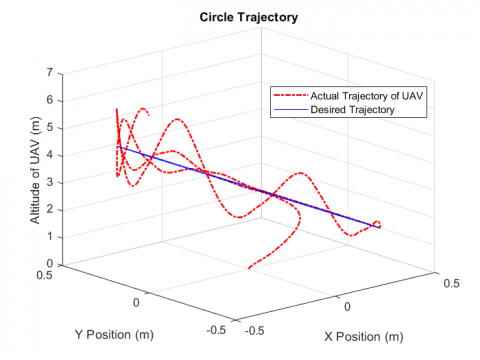(a) The 3D simulation of the quadrotor in circle trajectory

## 2.2.png(b) The dynamic response details for Z position in circle trajectory

## 2.3.png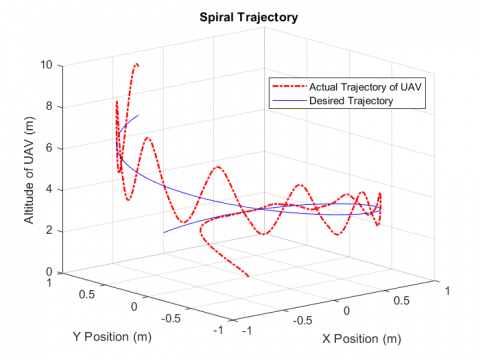(c) The 3D simulation of the quadrotor in a spiral trajectory

## 2.4.png(d) The dynamic response details for Z position in a spiral trajectory

## 2.5.png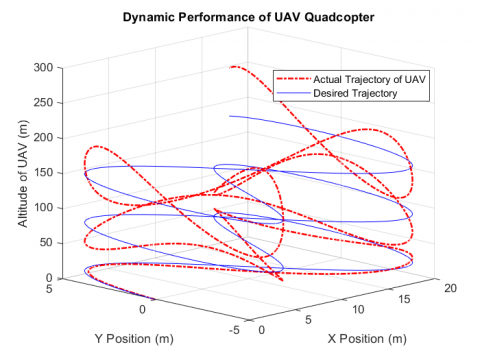(e) The 3D simulation of the quadrotor in lemniscate trajectory

## 2.6.png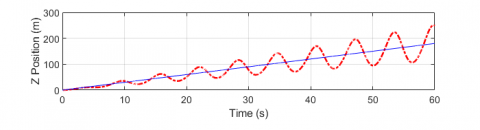(f) The dynamic response details for Z position in a lemniscate trajectory

Figure 2. The quadrotor’s dynamics response in the presence of external disturbances using SMC control (A=B=C=D=0.8)

Then, the NN algorithm is implemented into the SMC controller, becoming the SMC-NN controller. The SMC-NN controller is a combination of the SMC controller and backpropagation NN algorithm. To validate this SMC-NN controller, the simulation is conducted under the same condition as the SMC controllers.

Figure 3 (a)-(f) shows the performance of quadrotor’s dynamics in various trajectories such as circle, spiral, and lemniscate trajectory, in the absence of time-varying external disturbances using SMC-NN controller. In the beginning, the circle trajectory is conducted to the quadrotor system. From Figure 3 (a), it can be seen that the quadrotor can track the circle trajectory without any chattering issues. However, there is a delay time that occurs in the first 30s of the simulation at the Z axes movement in achieving the desired trajectory, as shown in Figure 2 (b).

Therefore, the simulation is conducted with the spiral and lemniscate trajectory that is implemented in the quadrotor’s system as shown in Figure 3 (c) and Figure 3 (e). Similar to a circular movement, in spiral and lemniscate movement, the quadrotor shows good performance without any chattering issues, but still there is a delay time at the first 30s of simulation in the Z axes movement, as shown in Figure 2 (d), in achieving the desired trajectory. Unlike the circle and spiral movement, the lemniscate movement shows a huge delay time as shown in Figure 3 (f) in the Z axes in achieving the desired trajectory.

## 3.1.png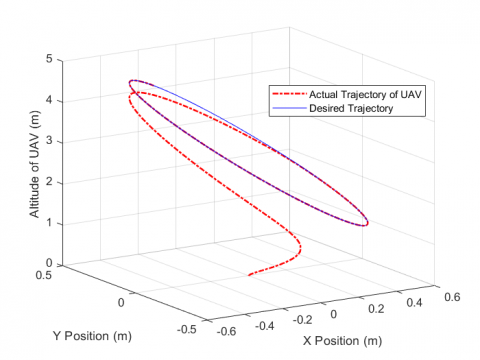(a) The 3D simulation of the quadrotor in circle trajectory

## 3.2.png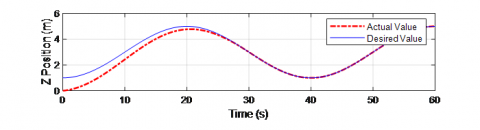(b) The dynamic response details for Z positions in circle trajectory

## 3.3.png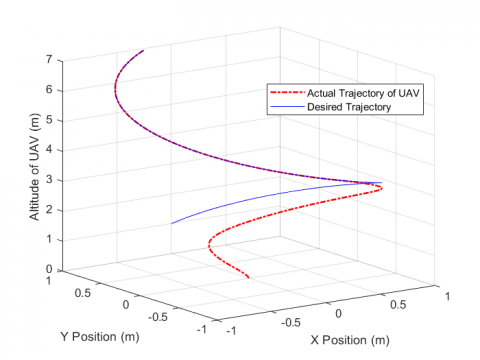(c) The 3D simulation of the quadrotor in a spiral trajectory

## 3.4.png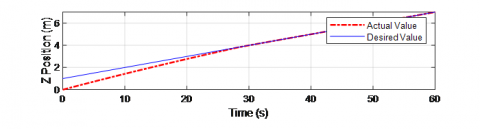(d) The dynamic response details for Z position in a spiral trajectory

## 3.5.png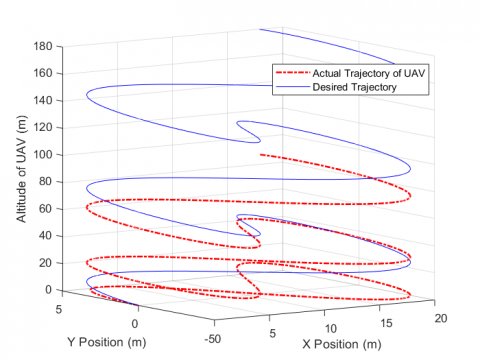(e) The 3D simulation of the quadrotor in lemniscate trajectory

## 3.6.png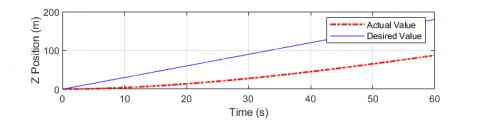(f) The dynamic response details for Z position in a lemniscate trajectory

Figure 3. The quadrotor’s dynamics response in the absence of disturbances using the SMC-NN control scheme (A=B=C=D=0)

The performance results as shown in Figure 3, indicates that the SMC-NN control scheme was able to control quadrotor in various trajectories in the absence of any disturbances. To examine how the further performance of the SMC-NN, the simulation is conducted to implement time-varying external disturbances into the quadrotor’s system with the gain value of 0.8, the same as the SMC’s simulation scenario.

The dynamic performances of the quadrotor using the SMC-NN controller in the presence of external disturbances are shown in Figure 4. The quadrotor’s system gets chattering issues in the Z axes at the first 30s of simulation in the presence of time-varying external disturbances, as shown in Figure 4 (a) and Figure 4 (b).

Similar to circle movement, the chattering issues also occur in spiral movement in the Z axes at the first 20s of the simulation as shown in Figure 4 (c) and Figure 4 (d). Unlike the others, the lemniscate movement is not showing any chattering issues as shown in Figure 4 (e) and Figure 4 (f). Finally, all the results above are summarized and the average duration of occurrence of oscillations during the flight is calculated, as shown in Table 4.

## 4.1.png(a) The 3D simulation of the quadrotor in circle trajectory

## 4.2.png(b) The dynamic response details for Z position in circle trajectory

## 4.3.png(c) The 3D simulation of the quadrotor in a spiral trajectory

## 4.4.png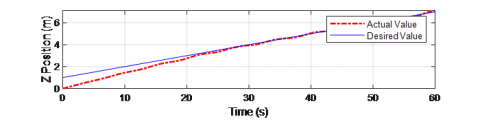(d) The dynamic response details for Z position in a spiral trajectory

## 4.5.png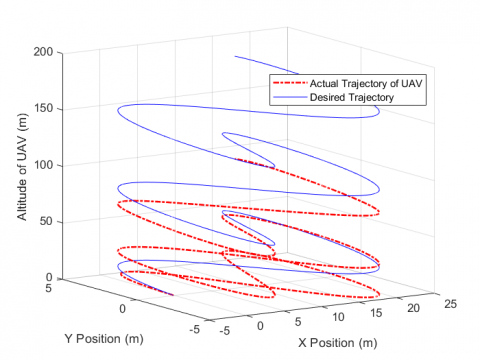(e) The 3D simulation of the quadrotor in lemniscate trajectory

## 4.6.png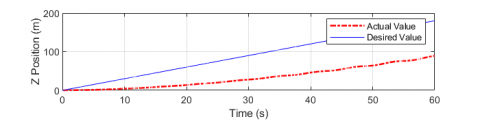(f) The dynamic response details for Z position in a lemniscate trajectory

Figure 4. The quadrotor’s dynamics response in the presence of disturbances using the SMC-NN control scheme (A=B=C=D=0.8)

Table 4. Quantitative comparison between SMC and SMC-NN controller based on the total time (s) of occurrence of oscillations in the presence of external disturbances (A=B=C=D=0.8)

 Trajectory Controller SMC SMC-NN Circle 52 s 10 s Spiral 51 s 9 s Lemniscate 54 s 25 s

Compared with the control scheme that only uses SMC as the main control as conducted by , and also by the simulation conducted in this study, as shown in Figure 2 (a)-(f), the gain value of 0.8 will make the quadrotor’s system becomes more jerk and unstable. On the other hand, with the same gain value of external disturbances, the SMC-NN controller can decrease any oscillations or instability in the dynamic performance of the quadcopter during the flight in the presence of external disturbances, as shown in Figure 4 (a)-(f) and Table 4. This indicates that the combination of SMC-NN performs a good performance for quadrotor dynamics in the presence of external disturbances than the SMC controller only.

5. Conclusions

A combination of sliding mode control with the backpropagation of neural network is examined in this article on various trajectories and several conditions in the absence and the presence of external disturbances. Three different trajectories are used in this article to examine the further performance of the control scheme. Numerical simulations using MATLAB have been carried out to validate the effectiveness of the SMC-NN control scheme. The results show that the tracking performance and the ability to achieve the desired trajectory in the presence of external disturbances is better than a control scheme that only uses SMC as the main control system. However, delay time occurs for each performance in the quadrotor’s dynamic system. This method can be extended and implemented into a real experiment which will be considered in future work.

Acknowledgment

The authors gratefully acknowledge the contributions of the Instrumentation, Control, and Optimization Laboratory of Sepuluh Nopember Institute of Technology (ITS)’s team and parties for supporting any software and computer requirements to meet the needs of this research.

References

 Chen, Y., Zhang, G., Zhuang, Y., Hu, H. (2019). Autonomous flight control for multi-rotor UAVs flying at low altitude. IEEE Access, 7: 42614-42625. http://doi.org/10.1109/ACCESS.2019.2908205

 Hedjar, R., Al Zuair, M.A. (2019). Robust altitude stabilization of VTOL-UAV for payloads delivery. IEEE Access, 7: 73583-73592. http://doi.org/10.1109/ACCESS.2019.2919701

 Al-Madani, B., Svirskis, M., Narvydas, G., Maskeliūnas, R., Damaševičius, R. (2018). Design of fully automatic drone parachute system with temperature compensation mechanism for civilian and military applications. Journal of Advanced Transportation, 2018: 2964583. http://doi.org/10.1155/2018/2964583

 Ali, Z., Chaudhry, S.A., Ramzan, M.S., Al-Turjman, F. (2020). Securing smart city surveillance: A lightweight authentication mechanism for unmanned vehicles. IEEE Access, 8: 43711-43724. http://doi.org/10.1109/ACCESS.2020.2977817

 Motlagh, N.H., Bagaa, M., Taleb, T. (2017). UAV-based IoT platform: A crowd surveillance use case. IEEE Communications Magazine, 55(2): 128-134. http://doi.org/10.1109/MCOM.2017.1600587CM

 Popescu, D., Stoican, F., Stamatescu, G., Ichim, L., Dragana, C. (2020). Advanced UAV–WSN system for intelligent monitoring in precision agriculture. Sensors, 20(3): 817. http://doi.org/10.3390/s20030817

 Murugan, D., Garg, A., Singh, D. (2017). Development of an adaptive approach for precision agriculture monitoring with drone and satellite data. IEEE Journal of Selected Topics in Applied Earth Observations and Remote Sensing, 10(12): 5322-5328. http://doi.org/10.1109/JSTARS.2017.2746185

 Polo, J., Hornero, G., Duijneveld, C., García, A., Casas, O. (2015). Design of a low-cost Wireless Sensor Network with UAV mobile node for agricultural applications. Computers and Electronics in Agriculture, 119: 19-32. http://doi.org/10.1016/j.compag.2015.09.024

 Alotaibi, E.T., Alqefari, S.S., Koubaa, A. (2019). Lsar: Multi-UAV collaboration for search and rescue missions. IEEE Access, 7: 55817-55832. http://doi.org/10.1109/ACCESS.2019.2912306

 Zhang, J., Ren, Z., Deng, C., Wen, B. (2019). Adaptive fuzzy global sliding mode control for trajectory tracking of quadrotor UAVs. Nonlinear Dynamics, 97(1): 609-627. http://doi.org/10.1007/s11071-019-05002-9

 Doukhi, O., Lee, D.J. (2019). Neural network-based robust adaptive certainty equivalent controller for quadrotor UAV with unknown disturbances. International Journal of Control, Automation and Systems, 17(9): 2365-2374. http://doi.org/10.1007/s12555-018-0720-7

 Sierra, J.E., Santos, M. (2019). Wind and payload disturbance rejection control based on adaptive neural estimators: application on quadrotors. Complexity, 2019: 6460156. http://doi.org/10.1155/2019/6460156

 Pérez, I.C., Flores-Araiza, D., Fortoul-Díaz, J.A., Maximo, R., Gonzalez-Hernandez, H.G. (2014). Identification and PID control for a quadrocopter. In 2014 International Conference on Electronics, Communications and Computers (CONIELECOMP), pp. 77-82. http://doi.org/10.1109/CONIELECOMP.2014.6808571

 Li, J., Li, Y. (2011). Dynamic analysis and PID control for a quadrotor. In 2011 IEEE International Conference on Mechatronics and Automation, pp. 573-578. http://doi.org/10.1109/ICMA.2011.5985724

 Tosun, D.C., Işık, Y., Korul, H. (2015). LQR control of a quadrotor helicopter. New Developments in Pure and Applied Mathematics, pp. 247-252. http://doi.org/10.1049/joe.2018.8126

 Wang, Y.M., Liu, Q.Q. (2013). LQG control of unmanned aerial vehicles under communication constraints. TELKOMNIKA Indonesian Journal of Electrical Engineering, 11(9): 4871-4877. http://www.iaesjournal.com/online/index.php/TELKOMNIKA/article/view/2426.

 Fessi, R., Bouallègue, S. (2019). LQG controller design for a quadrotor UAV based on particle swarm optimisation. International Journal of Automation and Control, 13(5): 569-594. http://doi.org/10.1504/IJAAC.2019.101910

 Li, S., Wang, Y., Tan, J., Zheng, Y. (2016). Adaptive RBFNNs/integral sliding mode control for a quadrotor aircraft. Neurocomputing, 216: 126-134. http://doi.org/10.1016/j.neucom.2016.07.033

 Zhang, K., Shi, Y., Sheng, H. (2021). Robust nonlinear model predictive control based visual servoing of quadrotor UAVs. IEEE/ASME Transactions on Mechatronics, 26(2): 700-708. http://doi.org/10.1109/TMECH.2021.3053267

 Zhao, S., Ruggiero, F., Fontanelli, G.A., Lippiello, V., Zhu, Z., Siciliano, B. (2019). Nonlinear model predictive control for the stabilization of a wheeled unmanned aerial vehicle on a pipe. IEEE Robotics and Automation Letters, 4(4): 4314-4321. http://doi.org/10.1109/LRA.2019.2931821

 Zhang, J., Gu, D., Deng, C., Wen, B. (2019). Robust and adaptive backstepping control for hexacopter UAVs. IEEE Access, 7: 163502-163514. http://doi.org/10.1109/ACCESS.2019.2951282

 Ali, R., Peng, Y., Iqbal, M.T., Amin, R.U., Zahid, O., Khan, O.I. (2019). Adaptive backstepping sliding mode control of coaxial octorotor unmanned aerial vehicle. IEEE Access, 7: 27526-27534. http://doi.org/10.1109/ACCESS.2019.2901366

 Ríos, H., Falcón, R., González, O.A., Dzul, A. (2018). Continuous sliding-mode control strategies for quadrotor robust tracking: Real-time application. IEEE Transactions on Industrial Electronics, 66(2): 1264-1272. http://doi.org/10.1109/TIE.2018.2831191

 Zenteno-Torres, J., Cieslak, J., Davila, J., Henry, D. (2021). Sliding mode control with application to fault-tolerant control: Assessment and open problems. Automation, 2(1): 1-30. http://doi.org/10.3390/automation2010001

 Yang, X., Chen, J., Dang, Y., Luo, H., Tang, Y., Liao, C., Chen, P., Cheng, K.T. (2019). Fast depth prediction and obstacle avoidance on a monocular drone using probabilistic convolutional neural network. IEEE Transactions on Intelligent Transportation Systems, 22(1): 156-167. http://doi.org/10.1109/TITS.2019.2955598

 Indrawati, V., Prayitno, A., Kusuma Ardi, T. (2015). Waypoint navigation of AR. Drone quadrotor using fuzzy logic controller. TELKOMNIKA (Telecommunication Computing Electronics and Control), 13(3): 381-391. http://doi.org/10.12928/telkomnika.v13i3.1862

 Surriani, A., Arrofiq, M. (2017). Altitude control of quadrotor using fuzzy self tuning PID controller. In 2017 5th International Conference on Instrumentation, Control, and Automation (ICA), pp. 67-72. http://doi.org/10.1109/ICA.2017.8068415

 Jiao, R., Chou, W., Rong, Y., Dong, M. (2020). Anti-disturbance control for quadrotor UAV manipulator attitude system based on fuzzy adaptive saturation super-twisting sliding mode observer. Applied Sciences, 10(11): 3719. http://doi.org/10.3390/app10113719.

 Kim, B.M., Choi, K.C., Kim, B.S. (2007). Trajectory tracking controller design using neural networks for tiltrotor UAV. In AIAA Guidance, Navigation and Control Conference and Exhibit, 2: 1653-1669 http://doi.org/10.2514/6.2007-6460

 Nguyen, N.P., Mung, N.X., Thanh, H.L.N.N., Huynh, T.T., Lam, N.T., Hong, S.K. (2021). Adaptive sliding mode control for attitude and altitude system of a quadcopter UAV via neural network. IEEE Access, 9: 40076-40085. http://doi.org/10.1109/ACCESS.2021.3064883

 Alsmadi, Y.M., Utkin, V., Haj-Ahmed, M., Xu, L., Abdelaziz, A.Y. (2018). Sliding-mode control of power converters: AC/DC converters & DC/AC inverters. International Journal of Control, 91(11): 2573-2587. http://doi.org/10.1080/00207179.2017.1390263

 Mobayen, S. (2018). Design of novel adaptive sliding mode controller for perturbed Chameleon hidden chaotic flow. Nonlinear Dynamics, 92(4): 1539-1553. http://doi.org/10.1007/s11071-018-4145-x

 Du, H., Yu, X., Chen, M.Z., Li, S. (2016). Chattering-free discrete-time sliding mode control. Automatica, 68: 87-91. http://doi.org/10.1016/j.automatica.2016.01.047

 Yang, Y., Yan, Y. (2016). Attitude regulation for unmanned quadrotors using adaptive fuzzy gain-scheduling sliding mode control. Aerospace Science and Technology, 54: 208-217. http://doi.org/10.1016/j.ast.2016.04.005

 Yin, Y., Niu, H., Liu, X. (2017). Adaptive neural network sliding mode control for quad tilt rotor aircraft. Complexity, 2017: 7104708. http://doi.org/10.1155/2017/7104708

 Razmi, H., Afshinfar, S. (2019). Neural network-based adaptive sliding mode control design for position and attitude control of a quadrotor UAV. Aerospace Science and Technology, 91: 12-27. http://doi.org/10.1016/j.ast.2019.04.055

 Bouadi, H., Bouchoucha, M., Tadjine, M. (2007). Sliding mode control based on backstepping approach for an UAV type-quadrotor. World Academy of Science, Engineering and Technology, 26(5): 22-27. https://doi.org/10.5281/zenodo.1077447

 Thanh, T.D.C., Ahn, K.K. (2006). Nonlinear PID control to improve the control performance of 2 axes pneumatic artificial muscle manipulator using neural network. Mechatronics, 16(9): 577-587. http://doi.org/10.1016/j.mechatronics.2006.03.011

 Xu, Q., Wang, Z., Zhen, Z. (2019). Adaptive neural network finite time control for quadrotor UAV with unknown input saturation. Nonlinear Dynamics, 98(3): 1973-1998. https://doi.org/10.1007/s11071-019-05301-1

 Zhang, J., Ren, Z., Deng, C., Wen, B. (2019). Adaptive fuzzy global sliding mode control for trajectory tracking of quadrotor UAVs. Nonlinear Dynamics, 97(1): 609-627. https://doi.org/10.1007/s11071-019-05002-9

 Mofid, O., Mobayen, S., Wong, W.K. (2021). Adaptive terminal sliding mode control for attitude and position tracking control of quadrotor UAVs in the existence of external disturbance. IEEE Access, 9: 3428-3440. https://doi.org/10.1109/ACCESS.2020.3047659